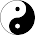Thursday, March 22, 2012

[Amazon Interview] Compare the BSTs

Given two unsorted integer arrays, your task is to compare the BST formed by both the arrays.

e.g [3 2 1 4 5] & [3 4 2 5 1]
when the BST is formed by taking the elements from the left, both BST turn out to be same.

3
/  \
2     4
/         \
1           5

Expected time complexity O(n).

Approach:
First observation is that the first element of the array forms the root of the BST.
So the first element needs to be equal of both the arrays.
Now the next left element which gets added to the BST is the next smaller element in the array, and same is true for the next right element in the BST.

So the sequence of the elements of the less than the the first element should be same in both arrays

And the same is true for the elements greater than the root element

So if the first array contains elements {9, 14, 8, 11, 4, 7, 15, 10, 6}
so any array which forms the same BST should have the elements of the sort
{9, ., 14, ..,11, .., 15, .., 10} & {9, .., 8, .., 4, .., 7, .., 6}

Both the preconditions can be verified trivially by two scans over the arrays.

1.Check if length of both arrays is same.
Check if root of both tree is same .
Partion the two arrays making root element i.e.array as pivot.
Now recursively check for left and right subtree.
We can use stable partitioning technique of Quicksort.

Thanx

2.In the above approach, we can use same arrays for keeping the partition elements
Space Complexity - O(1)
Worst case time complexity - O(n^2), if arrays make skewed BST.

3.@Navin ...
the average case O(nlogn) and worst case O(n^2) can be achieved naively by creating both the BST and comparing.

Try something else to reach a worst case time complexity of O(n)

4.#include
int Same_BST(int* arr1,int* arr2,int len1,int len2)
{
int i=0,j=0;
if(len1!=len2)//check length is same
return 0;
if(arr1[i]!=arr2[j])//root is same
return 0;
while(iroot) are same
{
if(arr1[i]>arr1)
{
while(arr2[j++]!=arr2[i]);
if(j>len2)
return 0;
}
i++;
}
i=0;
j=0;
while(ilen2)
return 0;
}
i++;
}
return 1;
}

5.#include
int Same_BST(int* arr1,int* arr2,int len1,int len2)
{
int i=0,j=0;
if(len1!=len2)//check length is same
return 0;
if(arr1[i]!=arr2[j])//root is same
return 0;
while(iarr1)
{
while(arr2[j++]!=arr2[i]);
if(j>len2)
return 0;
}
i++;
}
i=0;
j=0;
while(ilen2)
return 0;
}
i++;
}
return 1;
}

6.I m trying to paste my code and it is not getting paste correctly.

7.@ashish can u explain ur logic.

8.@aviral
let's take this eg:
e.g [3 2 1 5 6 4] & [3 5 2 4 1 6]
Your logic doesn't hold good. Correct me if i am wrong.

9.boolean check(int a[],int b[])
{
int less1[],less2[],grt1[],grt2[];
if(a!=b || a.length != b.length) return false;
for(int i=1,j=k=m=n=0,i<n;i++)
{
if(a[i]<a)
{ less1[j] = a[i]; j++; }
else { grt1[k] = a[i]; k++; }

if(b[i]<b[o])
{ less2[m] = b[i]; m++; }
else { grt2[n] = b[i]; n++; }
}

if(less1[] == less2[] && grt1[] == grt2[])
return true;
else return false;

}

10.If the breadth first traversal of the trees match then they are identical.